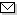Language:   Search:   Contact# Search and Browse the MSC 2000

Search:
Please note that in the second half of 2009, MSC2010 is deployed in the production of ZMATH and will become official standard in January 2010.

Enter a query and click »Search«...

11-XX Number theory → ZMATH
11-00 General reference works (handbooks, dictionaries, bibliographies, etc.) → ZMATH
11-01 Instructional exposition (textbooks, tutorial papers, etc.) → ZMATH
11-02 Research exposition (monographs, survey articles) → ZMATH
11-03 Historical (must also be assigned at least one classification number from Section 01) → ZMATH
11-04 Explicit machine computation and programs (not the theory of computation or programming) → ZMATH
11-06 Proceedings, conferences, collections, etc. → ZMATH
11Axx Elementary number theory {For analogues in number fields, see 11R04} → ZMATH
11Bxx Sequences and sets → ZMATH
11Cxx Polynomials and matrices → ZMATH
11Exx Forms and linear algebraic groups [See also 19Gxx] {For quadratic forms in linear algebra, see 15A63} → ZMATH
11Fxx Discontinuous groups and automorphic forms [See also 11R39, 11S37, 14-XX, 14Gxx, 14Kxx, 22Exx, 22E50, 22E55, 30F35, 32Nxx] {For relations with quadratic forms, see 11E45} → ZMATH
11Gxx Arithmetic algebraic geometry (Diophantine geometry) [See also 11Dxx, 14-XX, 14Gxx, 14Kxx] → ZMATH
11Hxx Geometry of numbers {For applications in coding theory, see 94B75} → ZMATH
11Kxx Probabilistic theory: distribution modulo \$1\$; metric theory of algorithms → ZMATH
11Lxx Exponential sums and character sums {For finite fields, see 11Txx} → ZMATH
11Mxx Zeta and \$L\$-functions: analytic theory → ZMATH
11Nxx Multiplicative number theory → ZMATH
11Pxx Additive number theory; partitions → ZMATH
11Rxx Algebraic number theory: global fields {For complex multiplication, see 11G15} → ZMATH
11Sxx Algebraic number theory: local and \$p\$-adic fields → ZMATH
11Txx Finite fields and commutative rings (number-theoretic aspects) → ZMATH
11Uxx Connections with logic → ZMATHAbel prize 2010I. M. Gelfand 1913-2009MSC2010